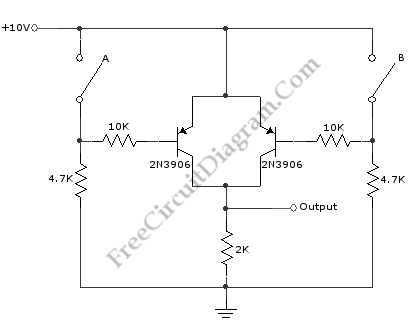# Basic Logic Gate with PNP TransistorThis schematic diagram shows a circuit of basic logic gate circuit. This PNP transistor circuit can act as OR gate or AND gate. If the input is closing of switch, this circuit acts as AND gate. This circuit acts as OR gate when the input is opening of switch. The phase inversion of input does not complicate overall circuitry. Here is the schematic diagram of the circuit: